# 使用matlab实现对winedataset的决策树（C4.5）构建及可视化

MATLAB R2019b

## dataset

1 - fixed acidity
2 - volatile acidity
3 - citric acid
4 - residual sugar
5 - chlorides
6 - free sulfur dioxide
7 - total sulfur dioxide
8 - density
9 - pH
10 - sulphates
11 - alcohol
Output variable (based on sensory data):
12 - quality (score between 0 and 10)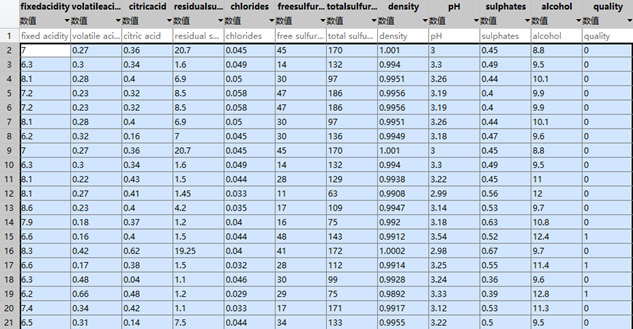http://archive.ics.uci.edu/ml/datasets/Wine+Quality
（顺便推荐一下这个网址，这是加利福尼亚大学的实验室数据集网站，里面也有很多其他非常优质的数据集）
（当然，如果下不下来可以评论里私聊~~）

## C4.5

（1） C4.5算法是决策树分类领域的一种较为经典的算法，下图展示了算法的流程：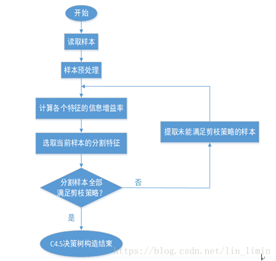（2）与ID3相比主要的改进：用信息增益率来选择属性。ID3选择属性用的是子树的信息增益，这里可以用很多方法来定义信息，ID3使用的是熵（entropy， 熵是一种不纯度度量准则），也就是熵的变化值，而C4.5用的是信息增益率。

（3）实现：
 数据预处理：

%随机采样的方法来分开训练样本和测试样本，并分开前11个属性值和第12列的label：
train_index=randperm(length(winedata),floor(length(winedata)/4*3));
traindata=winedata(train_index,:);
train_features=traindata(:,1:(size(traindata,2))-1)
train_targets=traindata(:,12)'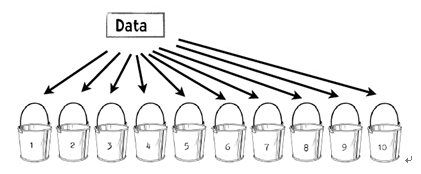（1）每一次迭代中留存其中一个桶。第一次迭代中留存桶1，第二次留存桶2，其余依此类推。
（2）用其他9个桶的信息训练分类器（第一次迭代中利用从桶2到桶10的信息训练分类器）。
（3）利用留存的数据来测试分类器并保存测试结果。

    testdata=csvread('ex6Data.csv',400*(i-1)+1,0,[400*(i-1)+1,0,400*i,11]);
if i==1
elseif i==10
else
traindata=[traindata1;traindata2];
end



 递归构造决策树

%计算当前节点的信息熵
Inode = -sum(Pnode.*log2(Pnode));
el= zeros(1, fea);
%记录每个特征的信息增益率
location= ones(1, fea)*inf;
for i = 1:fea
%遍历每个特征
info= sum(-node.*log(eps+node)/log(2));
%每个特征分别计算信息熵,eps是为了防止对数为1

el(i) = (Inode-sum(rocle.*info))/(-sum(rocle.*log(eps+rocle)/log(2)));
%信息增益率

[~, s] = max(I);  %求所有分割点的最大信息增益率
el(i) = spl(s);
location(i) = pe(s);
el(i) = spl(s);

location(i) = pe(s);
%对应特征i的划分位置就是能使信息增益最大的划分值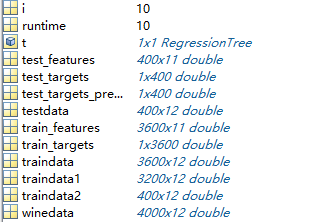 剪枝
%定义一个阈值(pruning)（这里为2），当达到某个节点的实例个数小于阈值时就可以停止决策树的生长。
if ((pruning > L) || (L == 1) ||(length(ale) == 1))
return

 计算准确率

accuracy(i,1)=cal_accuracy(test_targets,test_targets_predict1)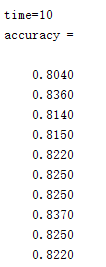如果我们取100的交叉验证，可以得到100次的准确率图表如下所示，可以看到分类准备率基本在0.8以上：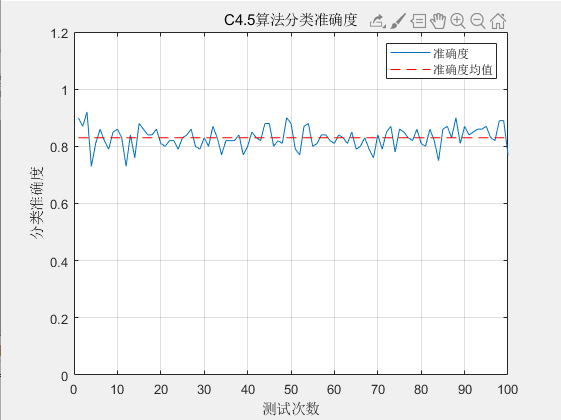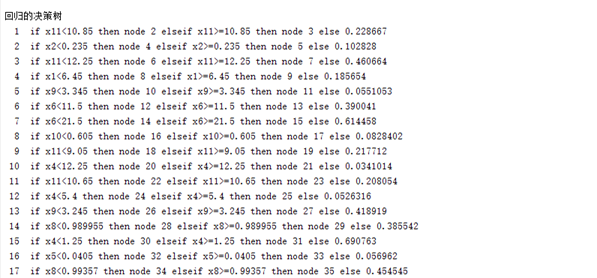https://ww2.mathworks.cn/help/stats/view-decision-tree.html

    view(t,'mode','graph')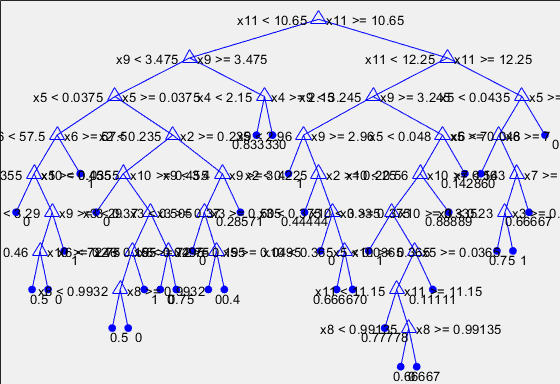部分剪枝以后：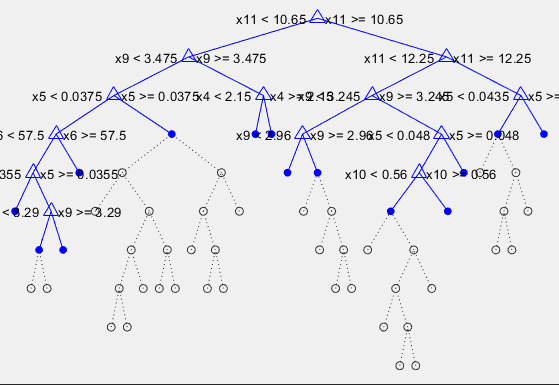代码：

clear;
clc;
% winedata=textscan('WineData.txt');
%

runtime=10;sum=0;
for i=1:runtime

%     tic;%用于计算程序运行时间

%train_index=randperm(length(winedata),floor(length(winedata)/4*3));%随机采样，3/4数据作为训练样本，其余的作为测试样本
%test_index=setdiff(linspace(1,length(winedata),length(winedata)),train_index);
%traindata=winedata(train_index,:);%训练样本
%testdata=winedata(test_index,:);%测试样本

if i==1
elseif i==10
else
traindata=[traindata1;traindata2];
end
train_features=traindata(:,1:(size(traindata,2))-1);
train_targets=traindata(:,12)';
test_features=testdata(:,1:(size(traindata,2))-1);
test_targets=testdata(:,12)';
test_targets_predict1 = C4_5(train_features', train_targets, test_features');  %调用C4.5算法用于分类

t=fitrtree(train_features,train_targets');%调用CART算法用于分类
view(t,'mode','graph')

%计算决策树预测的准确度
fprintf('time=%d',i)
accuracy(i,1)=cal_accuracy(test_targets,test_targets_predict1)
sum=sum+accuracy(i,1);
end
sum/10

plot(accuracy(:,1));
hold on;
plot(repmat(mean(accuracy(:,1)),length(accuracy(:,1)),1),'r--');
legend('准确度','准确度均值');
title('C4.5算法分类准确度');
xlabel('测试次数');
ylabel('分类准确度');
ylim([0,1.2]);
grid;



3、M = csvread(‘filename’, row, col, range)

（在这个的实现中，我个人觉得使用拼接比用并和交效果更好，因为数据集中存在这重复的数据）

https://jingyan.baidu.com/article/6525d4b1a97799ac7d2e94e9.html

https://blog.csdn.net/lin_limin/article/details/80998689（决策树）
https://www.cnblogs.com/BlameKidd/p/9735102.html（交叉验证

http://archive.ics.uci.edu/ml/
https://jingyan.baidu.com/article/6525d4b1a97799ac7d2e94e9.html（矩阵拼接）
https://jingyan.baidu.com/article/aa6a2c149ccf240d4c19c407.html（条件语句）
https://ww2.mathworks.cn/help/stats/view-decision-tree.html

03-11
07-11835805-091万+
08-102145
05-26616
09-292万+
04-094506
10-234万+
09-141万+
03-04